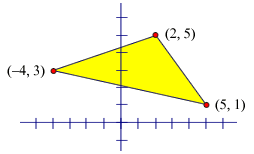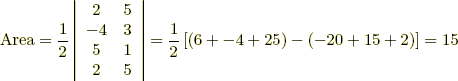# area of a convex polygon

Area of a Convex Polygon

The coordinates(x1, y1),(x2, y2),(x3, y3),. . . ,(xn, yn) of a convexpolygon are arranged in the "determinant" below. The coordinates must be taken in counterclockwise order around the polygon, beginning and ending at the same point.Example: Find the area of this polygon: